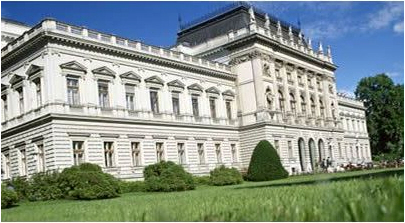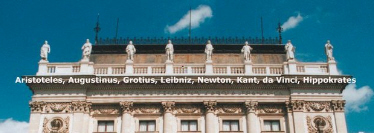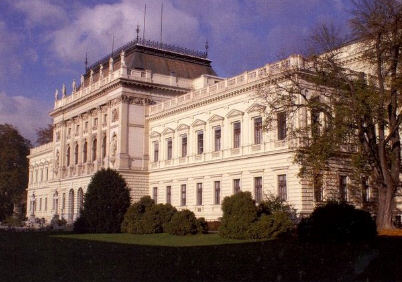# of 3-Class FieldsOriginal Problem. In the case of an odd prime p, algebraic number fields K with Hilbert p-class field tower K = Fp0(K) < Fp1(K) < Fp2(K) < … < Fpn(K) = Fp∞(K) of finite length Lp(K) = n bigger than two were unknown until August 24, 2012. This day was the historic event of the discovery of complex quadratic fields K = Q(D1/2) with 3-class field towers consisting of exactly three stages, that is L3(K) = 3. Modified Problem. All these fields had 3-class groups of type (3,3) and it remained an open problem whether there exist number fields K with non-elementary p-class group, and finite length Lp(K) > 2, for an odd prime p. It was the 5th of May, 2013, that we succeeded in providing evidence for the existence of 3-class field towers of exactly three stages over complex quadratic fields with 3-class groups of non-elementary type (3,9). Presentation of Recent Results of May 5, 2013. Let D denote the discriminant of a complex quadratic field K. We begin with arithmetical data whose investigation goes back to 2009 and 2010 , and was completed at the end of July 2011 . Punctured transfer kernel types (TKT) and transfer target types (TTT) were defined in a preprint 2011 . For D = -42567, the field K has punctured TKT C.4, (3,1,1;3), and homocyclic TTT [(9,9,9),(3,27),(3,27),(3,3,9)]. For D = -35331, the field K has punctured TKT C.4, (3,1,1;3), and heterocyclic TTT [(3,9,27),(3,27),(3,27),(3,3,9)]. For D = -116419, the field K has punctured TKT D.5, (2,1,1;3), and homocyclic TTT [(9,9,9),(3,27),(3,27),(3,3,9)]. For D = -17723, the field K has punctured TKT D.5, (2,1,1;3), and heterocyclic TTT [(3,9,27),(3,27),(3,27),(3,3,9)]. For D = -11651, the field K has punctured TKT D.10, (4,1,1;3), and TTT [(3,9,27),(3,27),(3,27),(3,3,9)]. For D = -31983, the field K has punctured TKT D.6, (1,3,2;1), and TTT [(3,27),(3,27),(3,27),(3,9,27)]. Now we present our new theorem, which links algebraic number theory with p-group theory via class field theory. In the sequel, we use the notation of the SmallGroups library and ANUPQ package of GAP and MAGMA for identifying p-groups and their descendants. Theorem. (Mayer) Let K be a complex quadratic field with 3-class group of type (3,9) and denote by G the Galois group Gal(F3∞(K)|K) of the maximal unramified pro-3 extension of K. If K has punctured TKT C.4, (3,1,1;3), and homocyclic TTT [(9,9,9),(3,27),(3,27),(3,3,9)], then its 3-tower group G is determined uniquely as <2187,168>-#2;2, a Schur σ-group of derived length 3, order 19683 and coclass 4, having 2nd derived quotient G/G'' ≅ <2187,168>-#1;2 of order 6561 and coclass 3. If K has punctured TKT C.4, (3,1,1;3), and heterocyclic TTT [(3,9,27),(3,27),(3,27),(3,3,9)], then its 3-tower group G is determined uniquely as <2187,168>-#2;6, a Schur σ-group of derived length 3, order 19683 and coclass 4, having metabelianization G/G'' ≅ <2187,168>-#1;6 of order 6561 and coclass 3. If K has punctured TKT D.5, (2,1,1;3), and homocyclic TTT [(9,9,9),(3,27),(3,27),(3,3,9)], then its 3-tower group G is determined uniquely as <2187,168>-#2;3, a Schur σ-group of derived length 3, order 19683 and coclass 4, having metabelianization G/G'' ≅ <2187,168>-#1;3 of order 6561 and coclass 3. If K has punctured TKT D.5, (2,1,1;3), and heterocyclic TTT [(3,9,27),(3,27),(3,27),(3,3,9)], then its 3-tower group G is determined uniquely as <2187,168>-#2;5, a Schur σ-group of derived length 3, order 19683 and coclass 4, having 2nd derived quotient G/G'' ≅ <2187,168>-#1;5 of order 6561 and coclass 3. If K has punctured TKT D.10, (4,1,1;3), and TTT [(3,9,27),(3,27),(3,27),(3,3,9)], then its 3-tower group G is either <2187,168>-#2;8 or <2187,168>-#2;9. Both are Schur σ-groups of derived length 3, order 19683 and coclass 4, having metabelianizations G/G'' ≅ <2187,168>-#1;8 resp. <2187,168>-#1;9. If K has punctured TKT D.6, (1,3,2;1), and TTT [(3,27),(3,27),(3,27),(3,9,27)], then its 3-tower group G is either <2187,181>-#2;4 or <2187,191>-#2;4. Both are Schur σ-groups of derived length 3, order 19683 and coclass 4, having 2nd derived quotients G/G'' ≅ <2187,181>-#1;4 resp. <2187,191>-#1;4. The proof is a consequence of the detailed table in � 1.2 of our data collection about descendant trees and Schur σ-groups . In fact, the table even determines the coclass tree and branch where G/G'' is located. Recall of Joint Results of August 24, 2012. To emphasize the similar structure of both theorems of May 5, 2013, and of August 24, 2012, we express the latter by means of the same concepts. Theorem. (Boston, Bush, Mayer) Let K be a complex quadratic field with 3-class group of type (3,3) and denote by G the Galois group Gal(F3∞(K)|K) of the maximal unramified pro-3 extension of K. If K has TKT E.6, (1,1,2,2), and TTT [(9,27),(3,3,3),(3,9),(3,9)], then its 3-tower group G is determined uniquely as <729,49>-#2;4, a Schur σ-group of derived length 3, order 6561 and coclass 3, having 2nd derived quotient G/G'' ≅ <729,49>-#1;5 = <2187,288> of coclass 2. If K has TKT E.14, (3,1,2,2), and TTT [(9,27),(3,3,3),(3,9),(3,9)], then its 3-tower group G is either <729,49>-#2;5 or <729,49>-#2;6. Both are Schur σ-groups of derived length 3, order 6561 and coclass 3, having metabelianizations G/G'' ≅ <729,49>-#1;6 = <2187,289> resp. <729,49>-#1;7 = <2187,290> of coclass 2. If K has TKT E.8, (2,2,3,4), and TTT [(3,9),(9,27),(3,9),(3,9)], then its 3-tower group G is determined uniquely as <729,54>-#2;4, a Schur σ-group of derived length 3, order 6561 and coclass 3, having metabelianization G/G'' ≅ <729,54>-#1;4 = <2187,304> of coclass 2. If K has TKT E.9, (2,3,3,4), and TTT [(3,9),(9,27),(3,9),(3,9)], then its 3-tower group G is either <729,54>-#2;2 or <729,54>-#2;6. Both are Schur σ-groups of derived length 3, order 6561 and coclass 3, having 2nd derived quotients G/G'' ≅ <729,54>-#1;2 = <2187,302> resp. <729,54>-#1;6 = <2187,306> of coclass 2. The proof is a consequence of the detailed table in � 1.1 of our data collection about descendant trees and Schur σ-groups . In fact, the table even determines the coclass tree and branch where G/G'' is located: branch B(6) of tree T(<243,6>) for the immediate descendants of <729,49>, and branch B(6) of tree T(<243,8>) for the immediate descendants of <729,54>. Let us illustrate the Boston-Bush-Mayer Theorem by arithmetical data whose investigation goes back to 2003 and 2004 , partially even to 1933 and 1982 . Transfer kernel types (TKT) and transfer target types (TTT) were defined in the related presentation . For D = -15544, the field K has TKT E.6, (1,1,2,2), and TTT [(9,27),(3,3,3),(3,9),(3,9)]. (TKT by Heider and Schmithals, 1982) For D = -16627, the field K has TKT E.14, (3,1,2,2), and TTT [(9,27),(3,3,3),(3,9),(3,9)]. (TKT by Heider and Schmithals, 1982) For D = -34867, the field K has TKT E.8, (2,2,3,4), and TTT [(3,9),(9,27),(3,9),(3,9)]. (Mayer, 2003) This is the unique capitulation type with 3 fixed points. The discriminant D lies below the limit -20000 of Angell's 1972 table, which was used by Heider and Schmithals in 1982. For D = -9748, the field K has TKT E.9, (2,3,3,4), and TTT [(3,9),(9,27),(3,9),(3,9)]. (Scholz and Taussky, 1933) This is the horribly obstinate field which caused 80 years of intolerable uncertainty, since Scholz and Taussky erroneously claimed that it has a 3-class field tower of length two. Nobody was able to confirm or refute this assertion. On August 23, 2012, Bush and Mayer disproved Scholz and Taussky's claim, using the concept of Schur σ-groups and providing evidence for a tower of length at least three. One day after, Boston, Bush and Mayer were even able to prove that the tower consists of exactly three stages. Important Alert. We point out that there are two kinds of descendant trees with respect to the Schur σ-cover of their metabelian vertices, that is, the set of all non-metabelian Schur σ-groups having a given vertex as their common metabelianization. Descendant trees with finite Schur σ-covers, in fact, containing a unique element, in all known examples. These trees consist of vertices with different TKT and contain a complete coclass tree with metabelian mainline. Examples are the trees with roots <729,49> (TKT c.18), <729,54> (TKT c.21) or <2187,168> (TKT d.10), <2187,181>, <2187,191> (both TKT e.14). Descendant trees with infinite Schur σ-covers. All vertices of these trees share a common TKT and only finitely many descendants are of the same coclass as the root. Examples are the trees with roots <729,45> (TKT H.4), <729,57> (TKT G.19) or <2187,173>, <2187,183> (both TKT B.7), <2187,178>, <2187,188> (both TKT E.12). For instance, <729,45>-#2;2 is only one of infinitely many Schur σ-groups G having the same metabelianization G/G'' ≅ <729,45>.* Web master's e-mail address: contact@algebra.at *